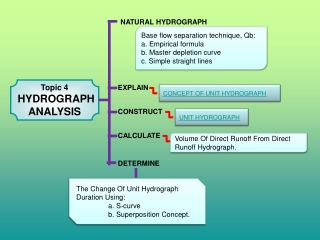DownloadDownload PresentationTopic 4 HYDROGRAPH ANALYSIS

# Topic 4 HYDROGRAPH ANALYSIS

Download Presentation## Topic 4 HYDROGRAPH ANALYSIS

- - - - - - - - - - - - - - - - - - - - - - - - - - - E N D - - - - - - - - - - - - - - - - - - - - - - - - - - -
##### Presentation Transcript

1. NATURAL HYDROGRAPH Base flow separation technique, Qb: a. Empirical formula b. Master depletion curve c. Simple straight lines Topic 4 HYDROGRAPH ANALYSIS EXPLAIN CONCEPT OF UNIT HYDROGRAPH CONSTRUCT UNIT HYDROGRAPH CALCULATE Volume Of Direct Runoff From Direct Runoff Hydrograph. DETERMINE • The Change Of Unit Hydrograph Duration Using: • a. S-curve • b. Superposition Concept.

2. HYDROGRAPH • A hydrograph is a graph showing the rate of flow (discharge) versus time past a specific point in a river, or other channel or conduit carrying flow. The rate of flow is typically expressed in cubic meters or cubic feet per second (cms or cfs).

3. DEFINITION OF NATURAL HYDROGRAPH • A natural hydrograph is one recorded at a stream gauging site and is a finger-print of the upstream drainage area’s response to rainfall

4. CONCEPT OF UNIT HYDROGRAPH • A Hydrograph is a graph showing changes in the discharge of river over a period of time. • It can also refer to a graph showing the volume of water reaching particular outfall or location in a sewerage network, graph are commonly used in the design of sewerage,more specillcally, the design of surface water sewegare systems and combination system.

5. a) A rising limb or concentration curve b) A peak or crest segment c) Falling limb or recession curve A hydrograph consists of:

6. The properties or characteristics of a unit hydrograph are a) the volume under a unit hydrograph is equal to 1 unit (1cm or 1in) rainfall excess b) If the duration of two rainfall excess events is equal, without regard to their respective rainfall intensities, they must result in the same hydrograph time base. c) The unit hydrograph results in a linear system whereby the direct runoff for a storm of a specified duration is directly proportional to the rainfall excess amount or volume d) Rainfall distribution for all equal duration storms is identical in space and time.

7. Calculate direct runoff from stream flow & base flow data Direct runoff = stream flow – base flow

8. Schedule below shows observation data from stream flow with base flow depth in catchment's area 250 km2. from that data , get direct runoff magnitude. example

9. Calculate Direct Runoff Depth Base On Volume DRO And Cathment Area. DRO Depth,rd = Vd A DIMANA; Vd = RUNOFF VOLUME =  Qn Δt A = CATCHMENT WIDE Qn = HYDROGRAPH ORDINATE Δt = HYDROGRAPH TIME INTERVAL

10. Calculate runoff volume and excess rain depth to a 7 acres catchment's area. Schedule below show a direct runoff data resultant from one precipitation EXAMPLE

11. Convert the duration of unithydrograph from short to long (superposition)

12. Combined 4hr-UH Lagged 4hr-UH 12hr-UH Convert The Duration Of UnitHydrograph From Short To Long (Superposition Method)- graphic The following are the ordinates of a 4-hour unit hydrograph. Derive the ordinates of a 12 - hour unit hydrograph 4hr-UH

13. Convert The Duration Of UnitHydrograph From Short To Long (Superposition Method)- table

14. Example : • The following are the ordinates of a 3-hour unit hydrograph. Derive the ordinates of a 6-hour unit hydrograph and plot the graph.

15. Solution

16. Question 1: • Determine the 4hr-UH from the 2hr-UH given in the table using the superposition principle method

17. Changing a Short Duration Unit Hydrograph to Long Duration using S-Curved method

18. Example • The ordinates of a 4-hour unit hydrograph for a particular basin are given below. Determine the ordinates of the S-curve hydrograph and there from the ordinates of the 6-hour unit hydrograph

19. Solution

20. Question 1 • Determine the 3hr-UH from the 1hr-UH given in the table using the S-curve method.

21. Changing a Long Duration Unit Hydrograph to Short Duration using S-Curved method

22. Question • The following are the ordinates of a 4hr – UH. Derive the ordinates of a 3hr-UH

23. Derive the S-curve for 4hr-UH

24. Hydrograph Unit from Stream Discharge Data

25. Example • Table below show runoff from hydrograph data, for a 50km2 catchment . Calculate the unit hydrograph (UH)10mm. Assume that the basic flow is 5.0m3/s

26. Solution

27. Mendapatkan Hidrograf Jumlah • Penggunaan UH10 bagi kawasan tadahan yang sama dengan contoh 1. • Diberikan ribut yang lain dengan tempoh yang sama bagi kawasan tadahan yang sama iaitu 50km2. Anggapkan aliran dasar 7 m3/s

28. Latihan • Cerapan kadaralir bagi satu kejadian hujan berkesan 12 jam bagi kawasan seluas 1150km2 adalah seperti yang ditunjukkan. Dapatkan hidrograf unit (UH)10mm untuk kawasan ini.

29. Diberi UH 4jam dan dapatkan UH -12jam dengan menggubakan kaeadah tindihan